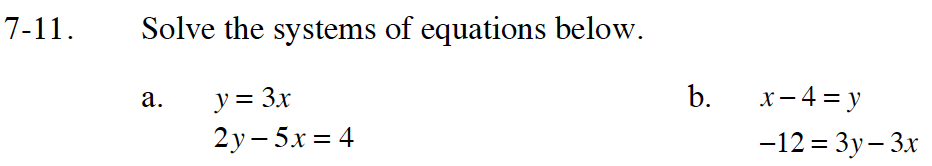Home > CC4 > Chapter 7 > Lesson 7.1.1 > Problem7-11

7-11.
1. Solve the systems of equations below. Homework Help ✎

1. y = 3x
2y – 5x = 4

2. x – 4 = y
–12 = 3y – 3xRead the Math Notes box in Lesson 6.2.3 to review the Substitution Method. Use it to solve both parts.

To start part (a) substitute 3x in for y into the second equation 2y − 5x = 4. So 2(3x) − 5x = 4.

Solve for x.

Once you solve for x, substitute that value in for x into one of the original equations: y = 3x or 2y − 5x = 4.
Solve for y.

Put the answer in point form (x, y).
The final answer is (4,12).

Substitute (x − 4) in for y in the second equation
-12 = 3(x-4) − 3x.

Follow steps 2 and 3 from part (a).

Read the Math Notes box in Lesson 6.4.1 to review the different types of solutions for systems of equations.